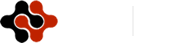American Journal of Information Science and Computer Engineering, Vol. 1, No. 1, May 2015 Publish Date: May 14, 2015 Pages: 1-9

Interference Management in MIMO-OFDM-Based Emerging Wireless Systems

Isiaka A. Alimi, Jide J. Popoola, Kayode F. Akingbade, Michael O. Kolawole

Department of Electrical and Electronics Engineering, School of Engineering and Engineering Technology, Federal University of Technology, Akure, Nigeria

Abstract

Despite the limited bandwidth resource and exponential growth in the number of subscribers, the requirement for high quality wireless communication coupled with higher data rates increases with various emerging multimedia applications. In the light of this, the challenge is managing the available spectrum efficiently. As such, an increase in the spectral efficiency implies ability to have greater throughput in the same amount of spectrum, and by implementing appropriate advanced technologies; the spectrum efficiency of wireless communication systems can be improved. It has been established that technologies such as orthogonal frequency division multiplexing (OFDM) and multiple input, multiple output (MIMO) systems, can enhance the performance of the emerging wireless communication systems significantly in terms of data rates and channel capacity in rich-scattering environments. To achieve the required efficiency, multi-user detection is of paramount importance in MIMO-OFDM systems to reduce signal interference such as multiuser interference. In this paper, both linear and nonlinear detection algorithms are broadly analysed and low-complex hybrid interference detection (HID) scheme is proposed. The proposed algorithm performance is estimated in terms of bit error rate (BER) and simulation results show near optimal performance.

Keywords

BER, HID, ICD, MUD, SIC, SQRD

1. Introduction

The need to build multiple networks to provide multiple services and meet subscribers’ requirements continues unabatedly as a result of various bandwidth-intensive applications and services. However, the downside is bandwidth limitation. To this effect, the spectrum efficiency needs to be enhanced by implementing advanced technologies , as well as channel interference reduction.

Studies including [2,3,4] have demonstrated that technologies such as orthogonal frequency division multiplexing (OFDM) and multiple input, multiple output (MIMO) systems, can enhance the performance of the existing wireless communication systems. In order to address this, the third generation partnership project (3GPP) has initiated activity on the long term evolution (LTE) of Universal Terrestrial Radio Access Network (UTRAN), which has enhanced performance than the existing wideband code division multiple access (WCDMA) based systems. The LTE is the radio technology designed to increase the capacity and speed of mobile networks [5,6]. The LTE uses Orthogonal Frequency Division Multiple Access (OFDMA) for the downlink and Single Carrier Frequency Division Multiple Access (SC-FDMA) for the uplink [5,7].

In an OFDM system, the entire channel is partitioned into many narrow parallel sub-channels and a block of data is modulated to a set of subcarriers . This increases the symbol duration and reduces the inter-symbol interference (ISI) caused by the multipath [7,8]. The MIMO systems implement multiple antennas at both sides of the link and have considerably higher spectral efficiency and have also improved the wireless communication systems capacity significantly [6,9].

There are different multi-user detection (MUD) schemes that can be employed in MIMO-OFDM systems to acquire the desired transmitted symbols while suppressing the multiple access interference. The detection schemes can be linear or nonlinear. The linear schemes, such as zero forcing (ZF) and minimum mean square error (MMSE), result in poor diversity performance . The nonlinear schemes, such as QR decomposition (QRD), successive interference cancellation (SIC) and parallel interference cancellation (PIC), are suboptimal and are prone to error propagation ; they involve iterative algorithms for removing the effects of the interfering users during each detection stage. On the other hand, the optimum maximum likelihood detection (MLD) scheme has the ability to offer the optimum BER (bit error rate) performance with full diversity but imposes computational complexity that increases exponentially with the number of simultaneous users [9,10,11].

This paper presents a low-complexity hybrid interference detection (HID) technique to optimise the performance of a MIMO-OFDM system. In this technique the MLD and inverse channel detector schemes are combined with the sorted QR decomposition (SQRD) and the successive interference cancellation (SIC) algorithms in order to achieve an optimal receiver. In the first stage of detection, MLD is employed in order to have the correct estimate of the symbol to be decoded so as to prevent error propagation associated with the suboptimal nonlinear schemes. The detection process by the MLD is limited to the second stage in order to reduce computational complexity related to the optimal schemes. Other stages of detection are accomplished by inverse channel detector schemes.

The rest of this paper is organized as follows:  Section 2 gives the broad overview of the multi-user detection (MUD) schemes. The mathematical description of the proposed novel hybrid interference detection (HID) scheme system model is presented in Section 3. Section 4 presents the performance curves generated based on simulation models developed in MATLAB® while Section 5 concludes this paper.

2. Overview of Multi-User Detection (MUD) Schemes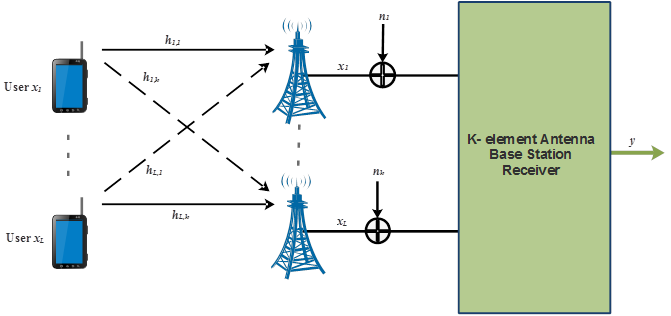Fig. 1. Multiuser MIMO-OFDM receiver model.

As a prelude, suppose Figure 1 represents a MIMO-OFDM system under flat fading, the complex baseband model can be expressed as:

y = Hx + n                                 (1)

where each user xi employs a single transmit antenna and transmits to one or several of the k-element receiver’s antennas yj, and contaminated by additive white Gaussian noise nj; where i = 1, 2, ..L; and j = 1, 2, ..k. The vectorial representations of the users signals, receivers, and noise are x  = [x1, x2, …, xL]T, y  = [y1, y2, …, yk]T  and n  = [n1, n2, …, nL]T, respectively. The symbol (.)T denotes transpose. The vector H of the channel matrix hLk is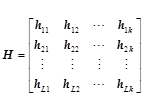(2)

As in this case, and in the general case, the channel matrix H is not necessarily square. The additive Gaussian white noise (AWGN) signal nk is assumed to exhibit a zero-mean and a variance of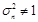. It is assumed that the signals transmitted by each user are independent of the other user’s, stationary, and having complex Gaussian distributed processes with zero-mean and unit variance (i.e.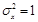).

The next paragraphs give an overview of the various multi-user detection (MUD) schemes that can be employed in a MIMO-OFDM system.

2.1. Maximum Likelihood (ML) Detection

The ML detector is the optimal detection method. It uses an exhaustive search for finding the most likely transmitted symbols. The ML detector with l simultaneous users and m number of bits per symbol has to invoke a total of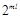metric evaluations in order to detect the l-user symbol vector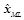that contains the most likely transmitted symbols of the l users of a specific subcarrier :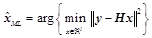(3)

where the set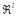constituted bynumber of trial vectors, which can be expressed as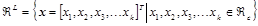(4)

and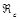denotes the set containing the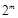number of legitimate complex constellation points associated with the specific modulation scheme employed. As a consequence, the MLD technique has disadvantage that its complexity grows exponentially with respect to the number of the transmit antennas for a given modulation. In order to reduce computational complexity, suboptimal linear receivers, such as zero forcing (ZF) and MMSE detectors, could be employed that allow an inversion of the channel matrix.

2.2. Zero Forcing (ZF) Linear Detection

The ZF linear detectors are inverse channel detector (ICD) that can be used to suppress the mutual interference between sub-streams. This implementation is accomplished by the normal inverse of square channel matrix, or by the Moore-Penrose pseudo-inverse of non-square matrix. Given that the channel matrix is not necessarily square, the Moore-Penrose pseudo-inverse is used, as such the "filtering" matrix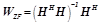(5)

where (.)H denotes the Hermitian transposition.

Detection of the transmitted symbols is optimally solved by the maximum likelihood scheme, i.e.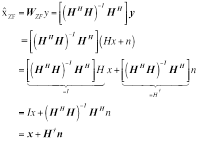(6)

where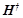is the Moore-Penrose pseudo-inverse of the channel matrix.

This implies that the ZF estimate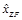consists of the decoded symbols plus a combination of the inverted channel matrix and the unknown noise vector. The disadvantage of ZF-scheme is that it would suffer from noise enhancement leading to suboptimal performance.

2.3. Minimum Mean Square Error (MMSE)

Unlike the ZF-method, the MMSE method attempts to minimize the mean-squared error between the estimated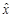and x, resulting in an optimal linear combination; i.e., putting noise into consideration, resulting in the following filtering matrix :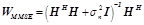(7)

where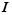is the identity matrix and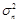is the additive white Gaussian noise (AWGN) variance for transmitted signal x. If the signal distribution variance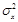is non-zero, we write the MMSE estimate as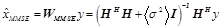(8)

Where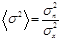(9)

At low signal-to-noise ratio (SNR), MMSE ﬁltering outperforms ZF ﬁltering; however at high SNR, MMSE ﬁlter approaches the ZF ﬁlter. The MMSE detector asymptotically reduces to Zero-Forcing detector when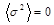, which, in this case, the MMSE detector can use the extended channel matrix,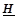for its filtering matrix, allowing its estimate to be written thus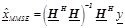(10)

where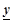is the extended received vector defined as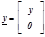(11)

and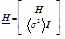(12)

Most linear interference cancellation techniques work on the principle that the desired layer is detected while considering other layers as interference. The nulling of each layer can be performed with a ZF or an MMSE equalizer. Subsequently, when the transmitted symbol vector has been detected, a decision on the vector is made either by quantization or by calculating the log-likelihood ratios (LLR) of the transmitted bits.

In general, the linear interference cancellation techniques, such as ZF and MMSE, have the disadvantage that some of the diversity potential of the receiver antenna array is lost in the decoding process. There are some nonlinear techniques such as ordered successive interference cancellation (OSIC) and parallel interference cancellation (PIC) that have been shown to have signiﬁcant performance improvements by taking the advantage of diversity potential of receive antennas . In latter cancellation technique, all interfering users are subtracted concurrently from the received signal while in the former technique; users are cancelled serially in the descending order of estimated received power that is from strongest to weakest .

2.4. Ordered Successive Interference Cancellation

V-BLAST  is a nonlinear successive interference cancellation (SIC) based detection algorithm with sorted cancellation ordering. In the SIC, signal components are detected in a sequential bias. For instance, in each data stream, the successively detected jth–stage symbol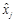is subtracted from the received signal, while the remaining received signal with the reduced interference is used for performing the signal estimation for the ensuing streams. This process continues until all transmitted symbols have been detected. If the detected symbol is obtained by the MMSE nulling matrix, then the MMSE estimate corresponding to each layer’s data stream is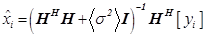(13)

The SIC receiver can suffer from error propagation if an incorrect detected symbol is used in the cancellation process .

In the ordered SIC (OSIC), post-detection signal-to-interference-plus-noise-ratio (SINR) is used to decide the order of detection For instance, the layer with the highest SINR is detected ﬁrst and its interference is cancelled from other streams. This process is prone to error propagation. To constrain the error propagation effect, it is desirable to detect reliable signals in the early stages; therefore, the detection nulling and cancellation order (NCO) will have significant influence on the performance of SIC methods. The MMSE criterion is considered and the post-detection SINR for each layer is maximized. For instance, suppose the received power at both antennas equivalent to the first transmitted symbol is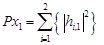(14)

where elements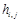are channel coefficients from the jth transmit to ith receive antennas and that of the received power at both antennas equivalent to the second transmitted symbol is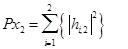(15)

If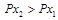, the receiver decides to eliminate effect of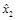from the received vector and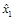will be re-estimated. The cancellation in descending order of signal strength is due to the fact that it is easier to acquire and perform demodulation on the strongest user . This enables high probability of making an accurate decision. Also, when the strongest user is removed, the multi-access interference (MAI) from it to other users will be eliminated. As the cancellation process continues, the weakest user will see a huge reduction in MAI and this will help in its detection.

2.5. Parallel Interference Cancelation

The parallel interference cancellation (PIC) scheme uses matched filter to estimate all the layers simultaneously. The estimates for each user can then be used to reduce the interference to and from the other signals by subtracting the estimate of each interferer from the desired user’s signal. This process eliminates all interference from the desired user. The PIC receiver process of cancellation compare to SIC scheme is faster and there is no delay incorporated at the receiver because cancellation is done concurrently. However, the receiver complexity is rather large compare to SIC scheme. Hence in order to obtain an optimal receiver performance, a trade-off between the computational time and receiver complexity is necessary . The QR decomposition detector can achieve this.

2.6. QR Decomposition Decoder

The QR decomposition decoder is a decoder that is based on linear algebra decomposition of the channel matrix H. The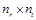channel matrix H is decomposed into a symmetrical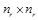dimensional orthogonal matrix (i.e. QTQ = I; unitary matrix)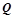and adimensional upper triangular matrix R. The decomposition of the channel matrix H is given by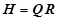(16)

If H is nonsingular, then this factorization is unique.

The sequence of detection is important because of the probability of error propagation. In the QR scheme, by permuting the columns of H prior to the decomposition can modify the sequence to improve performance. This process leads to different Q and R matrices. The method involves searching for the optimum R that maximizes the SNR in each step of the detection process. The computational effort involved in finding the optimum detection sequence can be reduced by adopting technique that can enhance performance of the QR decomposition scheme known as the sorted QR decomposition (SQRD).  The purpose of the SQRD is to sort the elements of main diagonal of R decreasingly in the order of evaluation of the decoding algorithm, that is, from the bottom right corner to the top left corner.

The SQRD algorithm performance is extremely close to that of OSIC but the SQRD algorithm always requires less computation effort to decode multiple antennas symbols compared to the OSIC decoder. Furthermore, performance of the SQRD scheme can be enhanced when implemented with the linear MMSE detector technique. In such a scheme (i.e. MMSE-SQRD), the extended channel matrixis QR decomposed is employed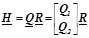(17)

Other detection technique like ZF-SQRD can also be employed but its performance is low compared to the MMSE-SQRD technique.

In general, these linear detection approaches to sorted QR decomposition do not always result in perfect detection of the sequence but in many cases the degradation is small compared to their reduced complexity. The computation requirement of the QR-based schemes is far simpler than the pseudo-inverse calculation required for the OSIC decoder. However, OSIC performance is relatively better .

3. Hybrid Interference Detection (HID) Scheme System Model

In this paper, a low-complex hybrid interference detection (HID) scheme for MIMO OFDM system is proposed. This (HID) scheme is formulated by combining the MLD and ICD schemes with the SQRD/OSIC algorithm in order to obtain an optimal receiver. By this approach, we attempt to integrate the trade-off between the computational time and receiver complexity, and ensure that the data estimates of the strongest user are cancelled at the first two stages of detection by employing the MLD scheme. This helps in having correct estimate of the symbol to be decoded and also prevent error propagation associated with the suboptimal nonlinear schemes. The detection process at MLD is limited to the second stage in order to prevent computational complexity related to the optimal schemes, whilst other stages of detection are accomplished by ICD schemes.

3.1. Implementation of HID with SQRD Schemes

The received symbol vector y is multiplied with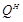prior to the symbol detection step to obtain an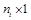modiﬁed received-vector c given by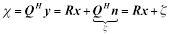(18)

With the fact that Q is a unitary matrix, the variance of the noise vector will not be affected. Since R is an upper triangular matrix, the kth element of c is expressed: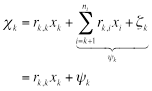(19)

In this process, the layer with the highest signal-to-interference-plus-noise ratio (SINR) is detected ﬁrst and its interference is cancelled from other streams using MLD in the decision-making. The location of the symbol on the triangular matrix R does not matter in the detection process. The decision statistic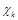is independent of the remaining transmit signals and can be used to find the estimate value of the layer with the highest SINR signal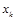defined as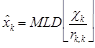(20)

This symbol is then used, by substitution, to detect the estimated value of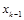given by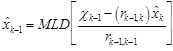(21)

Hence,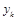is free of interference from layers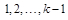. As interference is canceled in each step of detection process, diversity is increased and other elements of x can be detected.

3.2. Implementation of HID with OSIC Schemes

The implementation of the OSIC component of the hybrid scheme is similar to the MMSE-OSIC scheme discussed in Sec. 2.4. The MLD scheme discussed in (20) and (21) is carried out to determine the layer with the highest SINR. After obtainingby the MLD scheme, it is then weighted with its corresponding channel coefﬁcient and subtracted from the received signal vector like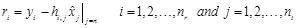(22)

So as to have a modiﬁed received signal vector, therefore, we have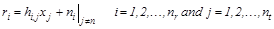(23)

The iteration will continue until all transmitted symbols have been detected.

4. Simulation Results and Discussions

The performances of different MIMO-OFDM based ICI cancellation schemes over frequency selective channels in terms of bit error rate (BER) are examined by simulations in this section. A Rayleigh channel is assumed with uncorrelated fading between the symmetric (2×2) antenna systems observed in λ/2-spaced uniform linear arrays. The information bits of each transmit chain are encoded with a ½-rate convolutional code. OFDM symbols employed are modulated by QPSK. The parameters used for the simulations are summarized in Table 1.

The performances of inverse channel detector (ICD) based systems are first considered. Figure 2 shows the BER of ZF, MMSE and MLD decoding. At a BER of 10−5 the difference between ZF and MMSE is approximately 3dB implying that the MMSE scheme performs better than the ZF scheme partly because of noise enhancement, as the noise variance of the system is not considered. The difference between MMSE and MLD decoding is about 25dB. This demonstrates that the ICD scheme performance is far below that of the MLD scheme.

Table 1. Simulations Parameters.

 Parameter Value Bandwidth 5 MHz Modulation type employed QPSK Channel coding ½-rate convolutional code Channel type Flat-fading Rayleigh channel Channel estimation Ideal Receiver decoder type ZF, MMSE, MLD, PIC, SIC, SQRD, HID IFFT size 512 OFDM cyclic prefix Normal Number of iterations 100000 No. of Receive antenna 2 No. of Transmit antenna 2

The performances of interference cancellation-based systems—PIC, SIC, ZF-SIC, MMSE-SIC and MLD—are evaluated and shown in Fig. 3. It is observed that integration of ICD schemes and SIC gives performance enhancement close to that of MLD scheme with MMSE-SIC having just about 1dB difference from that of MLD scheme at a BER of 10−5. This loss is due to the error propagation between data layers. It can be seen in Fig. 3 the relative advantage of considering the power levels of the received estimated symbols by the OSIC; and at a BER of 10−5, there is about 5dB difference between the conventional SIC and MMSE-SIC. Therefore, we can conclude that the scheme that takes order of power levels of the received estimated symbols into consideration is 5dB better than the conventional one because it is less prone to the error propagation due to incorrect detected symbol.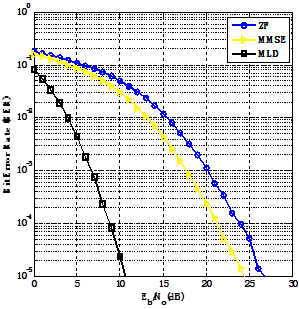Fig. 2. The performance analyses of the ICD and MLD schemes.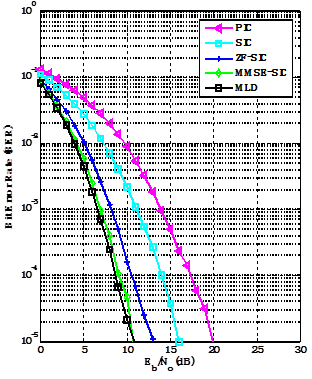Fig. 3. The performance analyses of the IC and MLD schemes.

Furthermore, the performances of QRD-based systems—QRD, SQRD, ZF-SQRD, MMSE-SQRD and MLD—are analysed, which Fig. 4 shows. It is seen that the integration of ICD schemes and SQRD also gives performance enhancement similar to that of SIC scheme but with less complexity. The MLD scheme gives about 2dB improvement over the MMSE-SQRD scheme at a BER of 10−5: this is due to suboptimal sorting. Moreover, the importance of sorting the columns of the QR decomposition scheme is seen in the improvement of about 6dB offers by the MMSE-SQRD over the conventional QRD.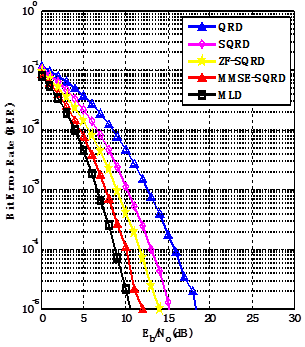Fig. 4. The performance analyses of the QRD and MLD schemes.

It is observed that performance of the MMSE-SIC is close to that of MLD, our proposed scheme is simulated and its performance compared with the MMSE-SIC and MLD schemes, as demonstrated in Fig. 5.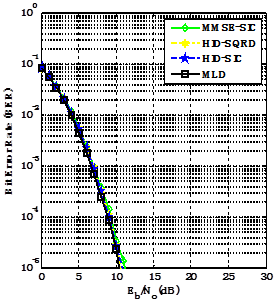Fig. 5. The performance analyses of the IC, MLD and the proposed scheme.

It is observed that performances of HID-SQRD and HID-SIC schemes are approximately equal to the MLD scheme at low SNR; however at high SNR, HID-SQRD and HID-SIC performances approach that of the MMSE-SIC scheme. Therefore, the proposed hybrid interference detection scheme has signiﬁcant performance improvements by taking the advantage of diversity potential of receive antennas as cancellation progresses. Figure 6 shows the plot of all analysed schemes.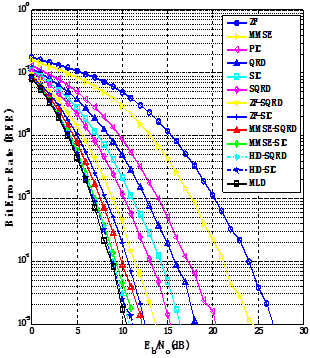Fig. 6. The performance analyses of different schemes considered.

5. Conclusions

The MIMO-OFDM transmission scheme offers increased data rate and higher spectral efficiency for the emerging wireless communication systems. There are different multi-users detection schemes that are applicable in wireless communication systems to achieve the target data rate and capacity. The schemes range from linear and nonlinear approaches. However, each scheme is prone to either poor performance or computational complexity. In this paper, a low-complexity and pragmatic hybrid interference detection (HID) scheme was proposed for the MIMO-OFDM systems and its performance compared with existing detection schemes. The proposed algorithm offers a good trade-off between acceptable degradation in the bit error rate (BER) performance and computational complexity. Also, the issue of error propagation associated with the SIC and SQRD has been addressed by the implementation of MLD scheme at the early stage of detection. The simulation results for different scheme are presented and the proposed scheme offers improved performance, and equals that of the MLD scheme at low SNR, while at high SNR its performance approaches that of the MMSE-SIC scheme. This behavior is similar to that of MMSE because at low SNR, MMSE ﬁltering outperforms ZF ﬁltering; however, at high SNR MMSE ﬁlter approaches that of the ZF ﬁlter.

References

1. H. Lee, B. Lee, and I. Lee, "Iterative detection and decoding with an improved V-BLAST for MIMO-OFDM systems", IEEE Journal on Selected Areas in Communications, vol. 24, no. 3, pp. 504-513, 2006.
2. I. A. Alimi, J. J. Popoola, K. F. Akingbade and M. O. Kolawole, "Performance analysis of bit-error-rate and channel capacity of MIMO communication systems over multipath fading channels", International Journal of Informatics and Communication Technology, vol. 2, no. 2, pp. 57-63, 2013.
3. V. S. Annapureddy, and V. V. Veeravalli, "Sum capacity of MIMO interference channels in the low interference regime", IEEE Transactions On Information Theory, vol. 57, no. 5, pp. 2565-2581, 2011.
4. C. Teekapakvisit, V. D. Pham, and B. Vucetic, "An Adaptive Iterative Receiver for Space-Time Coding MIMO Systems", Journal of Telecommunications and Information Technology, vol. 1, pp. 54-59, 2006.
5. J. Lee, J. K. Han, and J. Zhang, "MIMO technologies in 3GPP LTE and LTE-advanced", EURASIP Journal on Wireless Communications and Networking, vol. 2009, pp. 1-10, 2009.
6. V. Namboodiri, H. Liu and P. Spasojevic´, "Successive interference cancelation and MAP decoding for mobile MIMO OFDM systems and their convergence behavior", EURASIP Journal on Wireless Communications and Networking, vol. 311, pp. 1-12, 2012.
7. M. Konrad and W. Gerstacker, Interference robust transmission for the downlink of an OFDM-based mobile communications system, EURASIP Journal on Wireless Communications and Networking vol. 2008, pp. 1-14, 2008
8. V. J. Naveen, K. M. Krishna and K. R. Rajeswari, "Performance analysis of equalization techniques for MIMO systems in wireless communication", International Journal of Smart Home vol. 4, no. 4, pp. 47-63, 2010.
9. P. Hiral, S. Minal and A. Aniruddh, "Comparative study approach for MIMO-OFDM using spatial multiplexing techniques", International Journal of Advanced Engineering Research and Studies, vol. II, issue II, pp. 32-35, 2013.
10. M. Jiang and L. Hanzo, "Multiuser MIMO-OFDM for next-generation wireless systems", Proceedings of the IEEE, vol. 95, no. 7, pp. 1430-1469, 2007.
11. D. Chang and D. Guo, "Spatial-Division Multiplexing MIMO Detection Based on a Modified Layered OSIC Scheme," IEEE Transactions on Wireless Communications, vol.12, no.9, pp.4258-4271, 2013.
12. A. Paulraj, R. Nabar and D. Gore, Introduction to Space-Time Wireless Communications. Cambridge University Press, 2003.
13. P. W. Wolniansky, G. J. Foschini, G. D. Golden and R. A. Valenzuela, "V-BLAST: an architecture for realizing very high data rates over the rich-scattering wireless channel", URSI ISSSE’98, Pisa, Italy, pp. 295-300, 1998.

BiographyIsiaka Ajewale Alimi received B.Tech. (Hons) and M.Eng. in Electrical and Electronics Engineering respectively from Ladoke Akintola University of Technology, Ogbomoso, Nigeria in 2001, and the Federal University of Technology, Akure, Nigeria in 2010. He is a Lecturer in the Department of Electrical and Electronics Engineering, Federal University of Technology, Akure, Nigeria. He has published 3 refereed international journals. He has extensive experience in radio transmission, as well as in Computer Networking. His areas of research are in Computer Networking and Security, Advanced Digital Signal Processing and Wireless communications. He is a COREN registered engineer.Jide Julius Popoola received B. Eng. (Hons) and M.Eng. (Communication) degrees from the Federal University of Technology, Akure, Nigeria in 1999 and 2003 respectively and PhD from the School of Electrical and Information Engineering, University of the Witwatersrand, Johannesburg, South Africa in 2012. He is a Lecturer in the Department of Electrical and Electronics Engineering, Federal University of Technology, Akure, Nigeria. He has published over 10 refereed international journals and conference papers. He is a member of IEEE. His research interests are in signal fading mitigation, radio spectrum management and cognitive radio technology.Kayode Francis Akingbade received Master of Engineering (MEng) and PhD degrees in Electrical Engineering (Communication) from the Federal University of Technology, Akure, Nigeria in 2003 and 2011, respectively. He is a Lecturer in the Department of Electrical and Electronics Engineering, Federal University of Technology, Akure, Nigeria. He has published over 4 refereed international journals and conference papers. His research interests are in Biomedical Engineering and Satellite Communication.Michael O. Kolawole earns B. Eng. (Victoria University, Melbourne 1986) and PhD (UNSW, 2000) in electrical engineering, and Master of Environmental Studies, MEnvSt (Adelaide, 1989). He is concurrently LEAD Scholar and Professor of Electrical Engineering (Communication) at the Federal University of Technology, Akure Nigeria and Director of Jolade Consulting Company. Michael has published over 50 peer-reviewed papers, holds 2 patents, author of three books, and has overseen a number of operational innovations. His research interests are broadly in the areas of biomedical engineering, satellite communication engineering, radar systems and tracking, and remote sensing.
 Contents 1. 2. 2.1. 2.2. 2.3. 2.4. 2.5. 2.6. 3. 3.1. 3.2. 4. 5.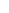600 ATLANTIC AVE, BOSTON,
MA 02210, USA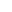+001-6179630233
JournalsJournal ListsAuthor GuidelinesPayment InformationSubmit a ManuscriptOpen Special IssuesJoin as Editorial MembersJoin as ReviewersPrivacy NoticeTerms of ConditionsHelp Center